• Save

# Missing Number Problems with Solutions for SSC and Bank Exams

2 months ago 29.0K Views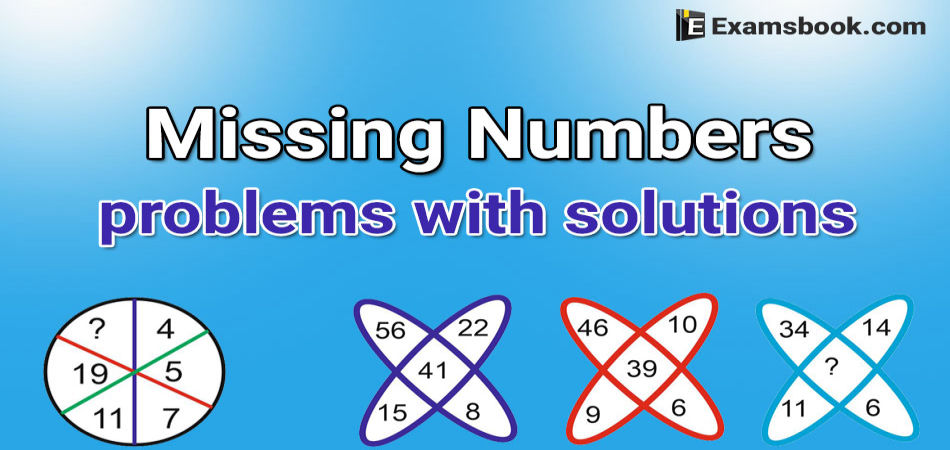In the competitive exam, missing number questions are also asked. So many students face difficulties in solving missing number problems because they don't understand question's sequence. So, here I am sharing missing number problems with solutions for ssc and bank exams.

You can learn how to solve missing number problems with different equations and examples. Try to solve these missing number problems yourself and increase your mental ability as well as your confidence.

You can more practice missing number questions and answers after learning how to solve problems.

Can solve also Missing Number Puzzles with Answers to obtain good marks in the competitive exams.

## Missing Number Problems with Solutions for Competitive Exams

Q.1. Find the missing number: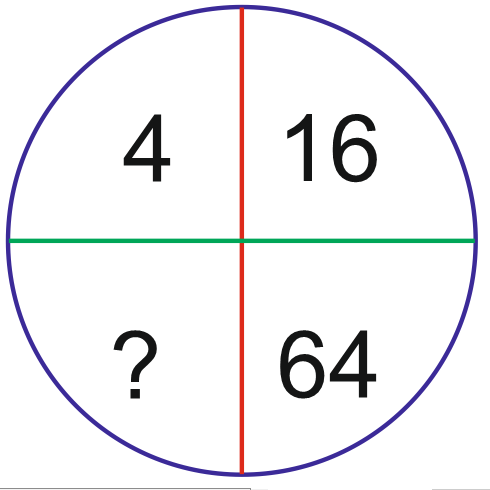(A) 16

(B) 52

(C) 112

(D) 256

Ans : D

Solution
4×4=16, 16×4=64, 64×4=256

Q.2. Find the missing number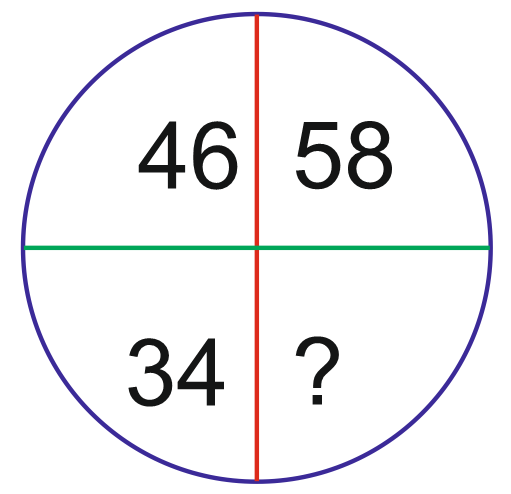(A) 92

(B) 72

(C) 22

(D) 68

Ans: C

Solution
This is a unique solution in which when we subtract 12 from given number, we get correct answer.

58 -12=46

46 -12=34

34 -12=22

Q.3. Find the missing number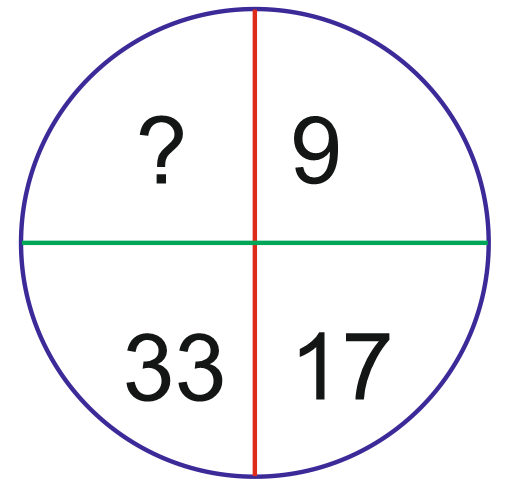(A) 60

(B) 68

(C) 55

(D) 65

Ans : D

Solution

This is a unique solution in which when we add multiple number of 8 in sequence.

9   + 8 = 17

17  + 16 = 33

33   + 32 = 65

Q.4. Find the missing number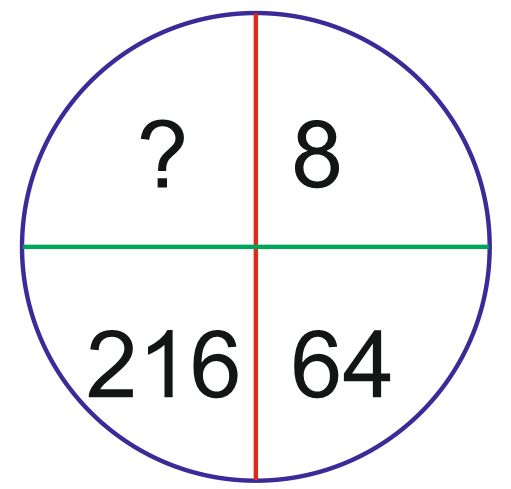(A) 343

(B) 512

(C) 729

(D) 1000

Ans : B

Solution
This is a unique solution in which we get cube root of multiple number of 2.

8 = (2)3

64 = (4)3

216 = (6)3

512 = (8)3

Q.5. Find the missing number

(A) 1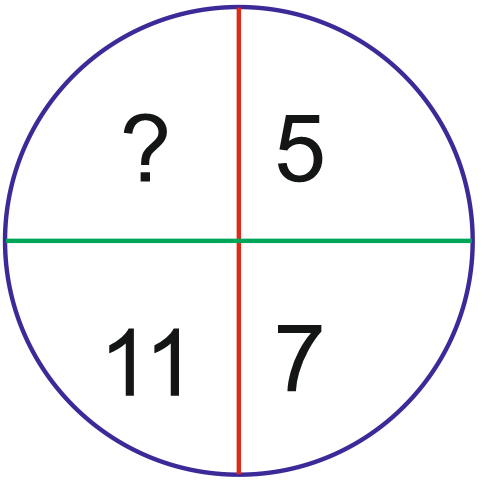(B) 9

(C) 12

(D) 17

Ans : D

Solution
5+2 = 7

7+4 = 11

⇒11+6 = 17

Q.6. Find the missing number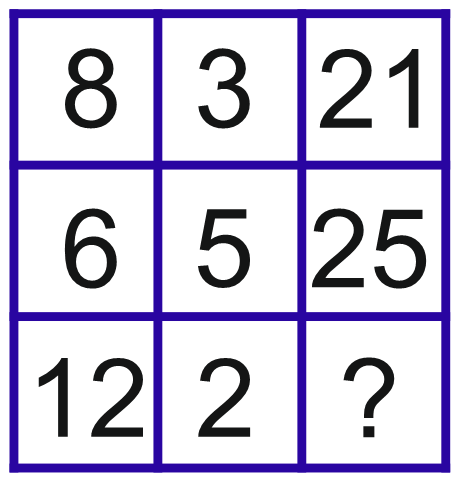(A) 24

(B) 19

(C) 22

(D) 20

Ans : C

Solution

8×3-3=24-3=21

6×5-5=30-5=25

12×2-2=24-2=22

Q.7. Find the missing number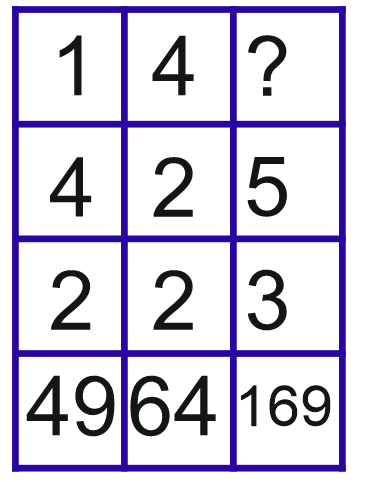(A) 3

(B) 6

(C) 5

(D) 4

Ans: C

Solution

As per :

(1+4+2) = (7)2=49

&(4+2+2) = (8)2=64

Similarly,

(?+5+3)3=169

?+5+3=13

?=13-8=5

Q.8. Find the missing number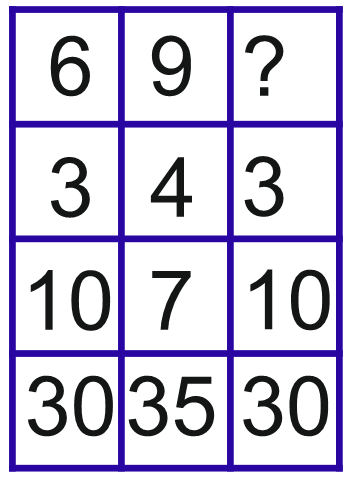(A) 9

(B) 6

(C) 7

(D) 0

Ans : B

Solution

In column I; (6-3)×10 =30

In column II; (9-4)×7=35

∴ In column III; (6-3)×10=30

Q.9. Find the missing number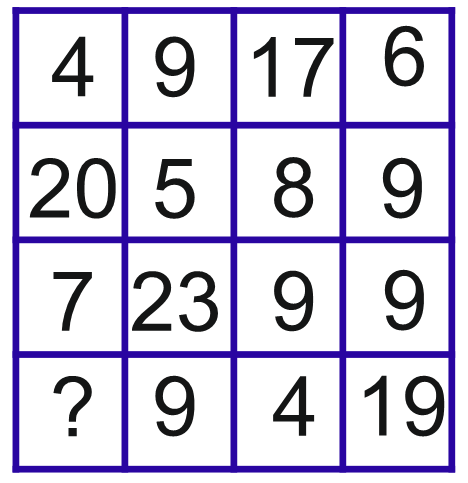(A) 7

(B) 9

(C) 8

(D) 6

Ans: C

Solution

1st row → 4+9+6-17=2,

2nd row → 5+8+9-20=2,

3rd row → 7+9+9-23=2,

4th row → 8+9+4-19=2,

∴ ? = 8

Q.10. Find the missing number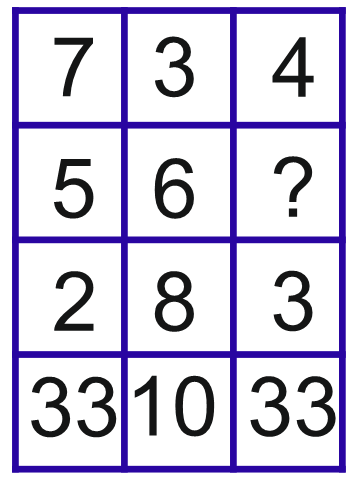(A) 7

(B) 8

(C) 9

(D) 26

Ans: C

Solution

In column I; 7×5-2=33

In column II; 3×6-8=10

In column III; 4×9-3=33

Keep practice these useful missing number problems solutions and ask me anything in the comment section if you have any query or doubt related missing number problems with solutions.

## About author

### Vikram Singh

Providing knowledgable questions of Reasoning and Aptitude for the competitive exams.

## Report Error : Missing Number Problems with Solutions for SSC and Bank Exams

Please Enter Message
Error Reported Successfully